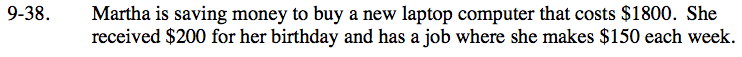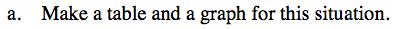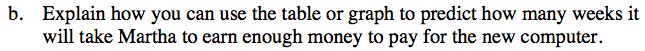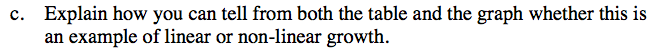### Home > CC3 > Chapter 9 > Lesson 9.1.3 > Problem9-38

9-38.
1. Martha is saving money to buy a new laptop computer that costs $1800. She received$200 for her birthday and has a job where she makes $150 each week. 9-38 HW eTool (Desmos). Homework Help ✎ 1. Make a table and a graph for this situation. 2. Explain how you can use the table or graph to predict how many weeks it will take Martha to earn enough money to pay for the new computer. 3. Explain how you can tell from both the table and the graph whether this is an example of linear or non-linear growth.Notice that Martha starts out with$200, and with each week she gains \$150.

Do you notice a pattern? Fill in the rest of the table.

Graph these points on your paper. Start at (0, 200). Draw a line through all the data points graphed.At which point on the graph does the amount of money Martha has equal the cost of the laptop computer?
How can you use the table to predict the amount of time until Martha has enough money?Linear growth is something increasing at a set rate. It is called linear growth because it forms a line when graphed.
Can you find a set rate of growth using your table? Does your graph form a line?

Use the eTool below to complete the problem.
Click the link at right for the full version of the eTool: CC3 9-38 HW eTool